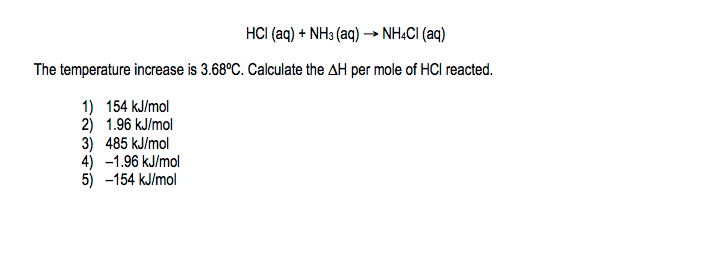# Problem: A 100.0 mL sample of 0.200 M aqueous hydrochloric acid is added to 100.0 mL of 0.200 M aqueous ammonia in a calorimeter whose heat capacity is negligible. The following reaction occurs when the two solutions are mixed.

###### FREE Expert Solution
93% (286 ratings)
###### Problem Details

A 100.0 mL sample of 0.200 M aqueous hydrochloric acid is added to 100.0 mL of 0.200 M aqueous ammonia in a calorimeter whose heat capacity is negligible. The following reaction occurs when the two solutions are mixed.Frequently Asked Questions

What scientific concept do you need to know in order to solve this problem?

Our tutors have indicated that to solve this problem you will need to apply the Constant-Pressure Calorimetry concept. You can view video lessons to learn Constant-Pressure Calorimetry. Or if you need more Constant-Pressure Calorimetry practice, you can also practice Constant-Pressure Calorimetry practice problems.

What is the difficulty of this problem?

Our tutors rated the difficulty ofA 100.0 mL sample of 0.200 M aqueous hydrochloric acid is ad...as high difficulty.

How long does this problem take to solve?

Our expert Chemistry tutor, Jules took 5 minutes and 5 seconds to solve this problem. You can follow their steps in the video explanation above.

What professor is this problem relevant for?

Based on our data, we think this problem is relevant for Professor Randles' class at UCF.